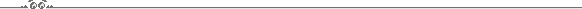# Boolean Circuit Complexity

## Fall Semester 1994/5The scribe notes of the course are still not in the state I would like them to be. They are unlikely to change however in the near future. I hope that even in the present form the notes can be of help to those looking for an introduction to circuit complexity. (March 22, 1995)• Lecture 1
• Basic concepts
• Post's theorem
• Shannon's counting argument
• Lecture 2
• Lupanov's construction
• Linear lower bounds on circuit size
• Lecture 3
• Simulation of Turing machines by circuits
• The complexity of arithmetical operations
• Lecture 4
• The relation between circuit depth and formula size
• The lower bound of Neciporuk
• Lecture 5
• The lower bound of Khrapchenko
• Shrinkage of de Morgan formulae under restriction
• The lower bound of Subbotovskaya
• The lower bound of Andreev
• Lecture 7
• Probabilistic circuits
• Lower bounds on monotone circuit size:
The method of approximations (Razborov's method)
• Lecture 8
• The method of approximations (continued)
• Lower bound for `is there a triangle?'
• Exponential lower bounds for clique functions
• Lecture 9
• Monotone versus non-monotone complexity
• Slice functions
• Depth and Communicaion complexity
• Lecture 10
• Lower bounds on Communication complexity
• A lower bound on the monotone depth of matching
• Lecture 11
• Bounded depth unbounded fanin circuits
• The representation of Boolean functions as integer polynomials
• Lecture 12
• Exponential lower bounds for parity
• The representation of Boolean functions as polynomials modulo 3
• Take-home exam